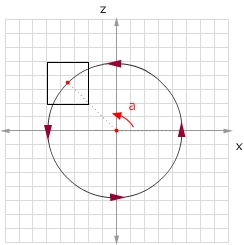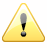## Flash与3D编程探秘（二）- 静态长方体

#### 动画制作步骤

1. 在这一个例子中，需要发挥一下空间想象力去定位长方体的顶点。至于场景上的网格，我想大家可以先把它放在场景的底部，等动画完成后再把它向上移动到合适的位置。

2. 开始和以前一样，定义原点以及焦距。另外也要初始几个常量。长方体围绕y轴公转，R是长方体公转的半径。

var PI = 3.1415926535897932384626433832795;

var R = 100;

var origin = new Object();
origin.x
= stage.stageWidth/2;
origin.y
= stage.stageHeight/2;
origin.z
= 0;

var focal_length = 300;
var angular_velocity = PI/180;
var _angle_xz = 0;

3. 接下来定义一个3D空间的点，长方体就围绕这个点进行公转。

var spin_center = new Object();
spin_center.x
= 0;
spin_center.y
= 0;
spin_center.z
= 100;

4. 创建一个场景舞台，用来盛放绘制的长方体。创建一个长方体的空Sprite并且把它添加到舞台上。

var scene = new Sprite();
scene.x
= origin.x;
scene.y
= origin.y
this.addChild(scene);

var box:Sprite
= new Sprite();
scene.addChild(box);

5. 下面需要一个函数来创建3D空间的点，那么下一次需要描述一个3D空间的点时，只需要执行这个函数，并且传入相应的x，y和z值即可。

function vertex(x, y, z):Object
{
var point3d
= new Object();
point3d.x
= x;
point3d.y
= y;
point3d.z
= z;

return point3d;
}

6. 你肯定猜到下一步要做的，那就是把一个3D空间的点转换成Flash能够理解的2D空间的点。转换的原理和第一节是一样的，这里就不解释了。

function convert(point3d, focal_length):Object
{
var point2d
= new Object();
var scale_ratio
= focal_length/(focal_length+point3d.z);
point2d.x
= point3d.x * scale_ratio;
point2d.y
= point3d.y * scale_ratio;

return point2d;
}

7. 下一步也是主要关心的循环函数。首先我想你明确这个函数要做的工作，那就是计算出长方体每个顶点的3D坐标，然后把它们转化成2D坐标，最后用Flash的绘制API建立图形。每一次函数执行，把长方体围绕y轴旋转的角度增加。然后根据这个角度，便可以计算出长方体中心点（对角线的交点）cente.x，center.y，center.z。很好，下面就可以确定这个长方体的每个点的坐标了。在这里我使用了一个边长为80正方体，你可以更改参数调试出你喜欢的长方体。

#### 注意function move(e:Event):void
{
var screen_points
= new Array();

_angle_xz
+= angular_velocity;

if (_angle_xz > 360)
{
_angle_xz
-= 360;
}

var center
= new Object();
center.x
= R*Math.cos(_angle_xz) + spin_center.x;
center.y
= 0 + spin_center.y;
center.z
= R*Math.sin(_angle_xz) + spin_center.z;

var points
= [
vertex(center.x
-40-40, center.z-40),
vertex(center.x
+40-40, center.z-40),
vertex(center.x
+40-40, center.z+40),
vertex(center.x
-40-40, center.z+40),

vertex(center.x
-4040, center.z-40),
vertex(center.x
+4040, center.z-40),
vertex(center.x
+4040, center.z+40),
vertex(center.x
-4040, center.z+40)
];

for (var i = 0; i < points.length; i++)
{
screen_points[i]
= convert(points[i], focal_length);
}

with (box.graphics)
{
clear();
lineStyle(.
50x0000001);
moveTo(screen_points[
0].x, screen_points.y);
lineTo(screen_points[
1].x, screen_points.y);
lineTo(screen_points[
2].x, screen_points.y);
lineTo(screen_points[
3].x, screen_points.y);
lineTo(screen_points[
0].x, screen_points.y);

moveTo(screen_points[
4].x, screen_points.y);
lineTo(screen_points[
5].x, screen_points.y);
lineTo(screen_points[
6].x, screen_points.y);
lineTo(screen_points[
7].x, screen_points.y);
lineTo(screen_points[
4].x, screen_points.y);

moveTo(screen_points[
0].x, screen_points.y);
lineTo(screen_points[
4].x, screen_points.y);
moveTo(screen_points[
1].x, screen_points.y);
lineTo(screen_points[
5].x, screen_points.y);
moveTo(screen_points[
2].x, screen_points.y);
lineTo(screen_points[
6].x, screen_points.y);
moveTo(screen_points[
3].x, screen_points.y);
lineTo(screen_points[
7].x, screen_points.y);
}
}

8. 最后在场景上添加一个循环执行事件。

this.addEventListener(Event.ENTER_FRAME, move);

#### 一个简单的Particle Sytem的例子作者：Yang Zhou 出处：http://yangzhou1030.cnblogs.com 本文版权归作者和博客园共有，转载未经作者同意必须保留此段声明。请在文章页面明显位置给出原文连接，作者保留追究法律责任的权利。

posted on 2008-10-30 07:29 yangzhou1030 阅读(...) 评论(...) 编辑 收藏